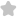Single Choice lim d"-3" If 0
Question# Single Choice lim d"-3" If 0 JEE/Engineering Exams Maths SolutionShare864.0 (1 ratings)( V ) ( frac{5}{8} leqslant cdot frac{0}{8}=frac{5}{8}= ) ( begin{array}{lll}frac{1}{8} & =end{array} ) ( sigma_{s}^{sigma}=underbrace{sigma}_{v}^{sigma}=s_{s}^{sigma} ) ( underbrace{sigma mid theta}_{1}, underbrace{theta P}_{+=}^{-1}} ) ( a: ) ( - )864.0 (1 ratings) Rate SolutionShareQuick and Stepwise Solutions Just click and SendOVER 20 LAKH QUESTIONS ANSWERED Download App for Free